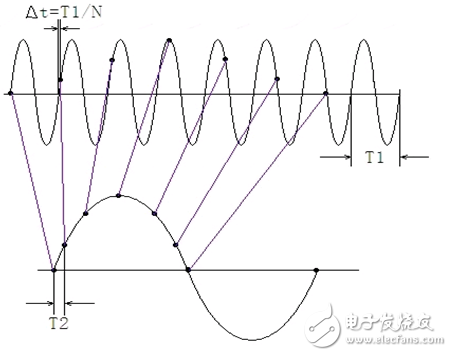# The sampling rate is lower than the bandwidth. Why can it be accurately measured?

High-bandwidth power analyzers on the market often have low sample rates, only one-half or less of the bandwidth. Is this really reasonable? Can I sample the input signal reliably? Can such sampling methods support high-precision electrical parameter measurements? What are the benefits of such a sampling method compared to high sampling rate sampling? This article will analyze the principles behind this phenomenon.

Sampling high frequency signals with a low and appropriate sampling rate has the following implications for the power analyzer:

1. Using the equivalent sampling principle to finely sample the high frequency signal, so that the sampled discrete signal has better phase resolution;

2. It is not necessary to use a high sampling rate ADC (the high sampling rate of the ADC means a reduction in the number of conversion bits).

Unlike an instrument such as an oscilloscope that uses a sampling rate higher than the bandwidth (the oscilloscope requires a high sampling rate to ensure the line of the restored waveform), the power analyzer uses a sampling rate lower than the bandwidth to ensure the accuracy and stability of the measurement results.

For high-frequency signals, from the sampling point of view, the accuracy and stability of the measurement results are: 1. phase uniformity and phase coverage density of the acquired signal; 2. equalization of the number of samples of each phase; 3. sampling points The number is sufficient. When measuring high-frequency signals, the two points 1 and 2 are more important. These two points must be guaranteed. The third point can also be satisfied when the sampling rate is lower than the bandwidth.figure 1

It can be seen that the equivalent sampling method samples only one point in one cycle of the input signal and the phase of sampling in each cycle has an increasing relationship (Î”t in Fig. 1), and adjusting the incremental phase can achieve the purpose of adjusting the phase resolution.

In addition, to ensure high-precision sampling, power analyzers typically use ADCs with higher conversion bits instead of higher sampling rates. Sampling accuracy is a very important basis for high-precision measurements. Because high-speed ADCs are often not ideal for other features, such as conversion bits, signal-to-noise ratio, and so on. With the equivalent sampling method, the power analyzer can eliminate the need to sacrifice high-precision signals for high-speed signals.

The power analyzer uses the equivalent sampling method for the high-frequency signal to ensure the above points. Therefore, even if the sampling rate is lower than the bandwidth, the high-frequency signal can be accurately measured.

40152 15Ah 3.2V Lithium Ion Battery

Lithium Polymer,Lithium Battery Types,Lithium Ion Car Battery,Hw40152S 15Ah Lifepo4 Battery

Zhejiang Xinghai Energy Technology Co.,Ltd , https://www.headwayli-battery.com